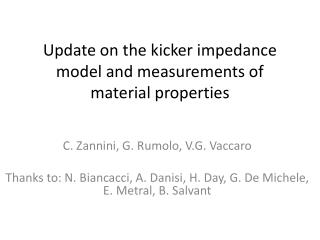Download PresentationUpdate on the kicker impedance model and measurements of material properties# Update on the kicker impedance model and measurements of material properties - PowerPoint PPT Presentation

Download Presentation##### Update on the kicker impedance model and measurements of material properties

Download Policy: Content on the Website is provided to you AS IS for your information and personal use and may not be sold / licensed / shared on other websites without getting consent from its author. While downloading, if for some reason you are not able to download a presentation, the publisher may have deleted the file from their server.

- - - - - - - - - - - - - - - - - - - - - - - - - - - E N D - - - - - - - - - - - - - - - - - - - - - - - - - - -
##### Presentation Transcript

1. Update on the kicker impedance model and measurements of material properties C. Zannini, G. Rumolo, V.G. Vaccaro Thanks to: N. Biancacci, A. Danisi, H. Day, G. De Michele, E. Metral, B. Salvant

2. Penetration depth in ferrite

3. Overview • Kicker impedance model • Wire measurements • Measurements of material properties

4. Simplified kicker model Courtesy T. Kroyer

5. Simulation models C-magnet model y x

6. Comparing the two models Real horizontal quadrupolar impedance calculated at x=1 cm Using the C-magnet model in the transverse horizontal impedance a high peak at 40MHz appears. At low frequency the Tsutsui model is not able to estimate correctly the kicker impedance. The two models are in good agreement at high frequency (>400MHz).

7. Comparing the two models Real longitudinal impedance We can see the peak also in the longitudinal and vertical impedance Real vertical detuning impedance calculated at y=0.5cm Impedance [Ohm]

8. C-magnet and Frame magnet C-magnet model y x Frame Magnet model

9. C-magnet and Frame magnet

10. Penetration depth in ferrite

11. Calculation of the impedance for the C-Magnet model Where is the low frequency impedance in a C-Magnet kicker model calculated using the SachererNassibian formalism and is the impedance calculated using the Tsutsui formalism. The Tsutsui impedance is calculated in H. Tsutsui. Transverse Coupling Impedance of a Simplified Ferrite Kicker Magnet Model. LHC Project Note 234, 2000. Instead we have to spend some word about the SachererNassibian impedance. This impedance is defined as: Where l is the length of the magnet, x0 the beam position, L the inductance of the magnet and Zg is the impedance seen by the kicker

12. Comparing with theoreticalresults Horizontal driving impedance calculated at x=1cm: MKP The simulations of the C-magnet model are in agreement with a theoretical prediction based on Sacherer-Nassibian and Tsutsui formalism Frame Magnet model Horizontal dipolar impedance

13. External circuits EK PSB The green curve depends from the cable properties (propagation and attenuation constants)

14. MKP: horizontal transverse impedance The segmentation seems to affect strongly the low frequency peak

15. Overview • Kicker impedance model • Wire measurements • Measurements of material properties

16. Measurements of the coupling impedance using the coaxial wire method The measured quantity is the transmission S21 cut-off frequency ≠0 cut-off frequency =0 TEM Propagationlosses Measured Losses = True Losses + Propagation losses For resonant structures:

17. Measurements of the coupling impedance using the coaxial wire method cut-off frequency =5.74 GHz Superfish

18. Overview • Kicker impedance model • Wire measurements • Measurements of material properties

19. Electromagnetic characterization of materials We characterize the material at high frequency using the waveguide method Coaxial line method

20. Status of the measurements We (me and G. De Michele) did measurements for some SiC in the ranges 10 MHz-2GHz and 8 - 40 GHz and for the ferrite 8C11 in the range 10MHz-10GHz The elaboration of the results will take at least one month (simulation time of the measurement setups)

21. Ferrite Model The ferrite has an hysteresis loop

22. The hysteresis effect in this measurements μ H In this measurements for the coaxial ferrite sample we have: